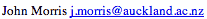Data Structures and Algorithms 7.4 Bin Sort

Assume that

1. the keys of the items that we wish to sort lie in a small fixed range and
2. that there is only one item with each value of the key.
Then we can sort with the following procedure:
1. Set up an array of "bins" - one for each value of the key - in order,
2. Examine each item and use the value of the key to place it in the appropriate bin.
Now our collection is sorted and it only took n operations, so this is an O(n) operation. However, note that it will only work under very restricted conditions.

#### Constraints on bin sort

To understand these restrictions, let's be a little more precise about the specification of the problem and assume that there are m values of the key. To recover our sorted collection, we need to examine each bin. This adds a third step to the algorithm above,
1. Examine each bin to see whether there's an item in it.
which requires m operations. So the algorithm's time becomes:
T(n) = c1n + c2m
and it is strictly O(n + m). Now if m <= n, this is clearly O(n). However if m >> n, then it is O(m).

For example, if we wish to sort 104 32-bit integers, then m = 232 and we need 232 operations (and a rather large memory!).
For n = 104:

nlogn ~ 104 x 13 ~ 213 x 24 ~ 217
So quicksort or heapsort would clearly be preferred.

An implementation of bin sort might look like:

```#define EMPTY	-1 /* Some convenient flag */
void bin_sort( int *a, int *bin, int n ) {
int i;
/* Pre-condition: for 0<=i<n : 0 <= a[i] < M */
/* Mark all the bins empty */
for(i=0;i<M;i++) bin[i] = EMPTY;
for(i=0;i<n;i++)
bin[ a[i] ] = a[i];
}

main() {
int a[N], bin[M];    /* for all i: 0 <= a[i] < M */
.... /* Place data in a */
bin_sort( a, bin, N );

```

If there are duplicates, then each bin can be replaced by a linked list. The third step then becomes:

1. Link all the lists into one list.
We can add an item to a linked list in O(1) time. There are n items requiring O(n) time. Linking a list to another list simply involves making the tail of one list point to the other, so it is O(1). Linking m such lists obviously takes O(m) time, so the algorithm is still O(n+m).

In contrast to the other sorts, which sort in place and don't require additional memory, bin sort requires additional memory for the bins and is a good example of trading space for performance.

 Although memory tends to be cheap in modern processors - so that we would normally use memory rather profligately to obtain performance, memory consumes power and in some circumstances, eg computers in space craft, power might be a higher constraint than performance.

Having highlighted this constraint, there is a version of bin sort which can sort in place:

```#define EMPTY	-1 /* Some convenient flag */
void bin_sort( int *a, int n ) {
int i;
/* Pre-condition: for 0<=i<n : 0 <= a[i] < n */
for(i=0;i<n;i++)
if ( a[i] != i )
SWAP( a[i], a[a[i]] );
}
```
However, this assumes that there are n distinct keys in the range 0 .. n-1. In addition to this restriction, the SWAP operation is relatively expensive, so that this version trades space for time.

The bin sorting strategy may appear rather limited, but it can be generalised into a strategy known as Radix sorting.

©, 1998